# Speed and Velocity

• Last Updated : 24 Jun, 2021

Mechanics can be termed as the branch of physics that is concerned with the concepts of energy and forces and their effect on bodies. It governs the relationships related to the motion of objects, that is, between matter, force, and its associated energy. It is responsible for the motion of bodies and the action of forces on these bodies as well. Practically, mechanics involve the design, construction, or operation of machines or tools. For instance, the distance covered by a car to stop traveling at a speed of 30 km/h.

The branch of classical mechanics concerned with the motion of points, object groups as well its systems, holding no reference to the motion of these objects, is called Kinematics, often termed as the “Geometry of Motion.

The sub-branch of physical science which is related to the motion of material objects under the effect of the physical factors affecting them, that is force, mass, momentum, energy is called the Dynamics.

Rest

Anybody is said to be at a rest position if there is no change in its position (distance, displacement) with time, with respect to its surroundings or a reference point.

Motion

The change in the position of anybody with respect to time can be termed as motion. Any object under motion can be visualized by the naked eye by determining the change in the positional coordinates and then, associating it through the eye of the arbitrary observer. Motion can be computed in terms of both the position vectors, that is, the displacement, distance, and taking into consideration the speed factors, that is, velocity, acceleration, speed, and time.

For instance, a spring ball attached to one end of a rod swinging at different time frames can be assumed to be in motion.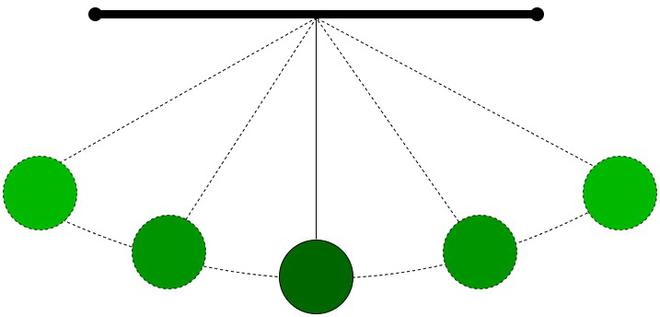A rubber ball undergoing displacement under the influence of motion

Difference in Scalar and Vector:

Distance

Distance is the complete length of the path between any two successive points. The distance is a scalar quantity, with only magnitude and no associated direction. The distance is denoted by the symbol ‘d’. Distance of any object is considered to be a positive commodity. Distances can be measured both along the straight as well as zig-zag paths. Distance of a body gives the detailed route information that is followed while travelling from one point to another.

Distance = Speed x Time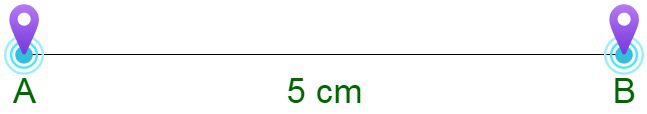Distance from location A to location B is 5 cm

Displacement

Displacement is the direct length of the minimum path between any two successive points. The displacement is a vector quantity, with both an associated magnitude and direction. It is denoted by ‘s’. The displacement of an object between any two points is considered to be positive, negative and even zero. Displacement is independent of the path and it only depends upon the initial and final position of the body. Therefore, it does not provide complete information on the route. Displacement is always indicated with an arrow.

Displacement = Velocity x Time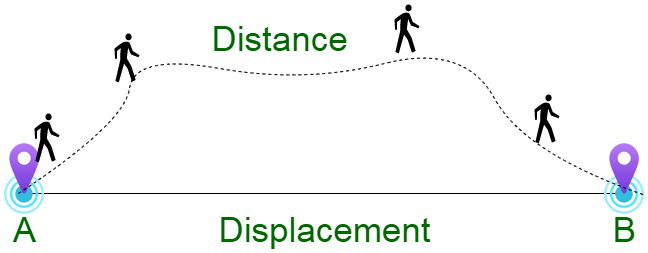Speed

Speed can be defined as the rate of change of position of an object moving in any direction. Speed is measured as the ratio of the distance covered by an object to the time in which the distance was covered. Speed of any body is considered to be a scalar quantity, with only magnitude and no associated direction.where, ‘s’ is the speed in m/s, ‘d’ is the distance traveled in m and t is the time taken in seconds.

Dimensional formula for Speed

Mathematically,Dimensional formula for Distance = M0L1T0

Dimensional formula for time = M0L0T1

Therefore, dividing dimensional formula for distance by dimensional formula for time;Hence,

Dimensional formula for speed = ML1T-1

Velocity

Velocity of an object can be defined as the rate of change of the object’s position with respect to a frame of reference and time. The displacement is a vector quantity, with both an associated magnitude and direction. SI unit is meter per second (m/s). The velocity of an object can be positive, negative or even zero. If there is a change in magnitude or the direction in the velocity of a body the body is said to be accelerating.

Initial velocity describes the pace with which any object travels when gravity first applies force on the object, whereas, the final velocity is a vector quantity that describes the commodities of the speed and direction of a body in motion once it has reached its maximum acceleration.

Constant Velocity

Constant velocity can be termed as the motion in a straight line at a constant speed. Algebraically,

x = x0 + vt

where,

x0 represents the position of the object at

t = 0, and the slope of the line indicates the object’s speed.

The velocity can be positive or negative, and is indicated by the sign of our slope. This tells us in which direction the object moves.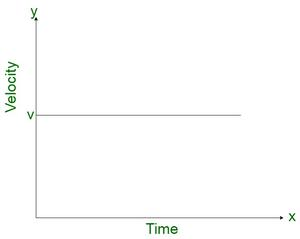Constant Velocity Graph

Velocity Units

The S.I unit of velocity is m/s (m/s).

Units and dimensions of velocity are as follows :

Difference between Speed and VelocitySpeed vs Velocity

Uniform Speed and Uniform Velocity

Variable Speed and Variable Velocity

Instantaneous Velocity and Instantaneous Speed

Instantaneous speed is always greater than or equal to zero, that is it is a positive commodity.  Instantaneous speed is a scalar quantity, associated with a magnitude and not direction. It is constant in case of uniform motion. It is a limit of the average speed for infinitely small time interval.

Instantaneous velocity is an indicator of how fast an object is travelling at different instants of time within a given time interval. Also termed as average velocity for a negligibly small time interval. To summarise, the instantaneous speed at any given time interval is equivalent to the magnitude of instantaneous velocity at that time.

We have,Here lim is taking operation of taking limit with time tending towards 0 or infinitely small. And,is differential coefficient – Rate of change of position with respect to time at an instant.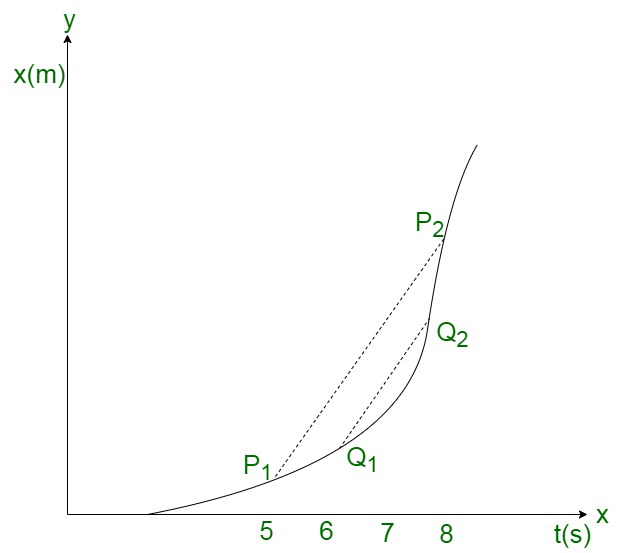P-T graph for Instantaneous Velocities

From the graph, we have,

Slope P1P2 – Velocity at an instant of 3 sec

Slope Q1Q2 – Velocity at an instant of 1 sec

Where as,

Instantaneous speed is referred as the magnitude of velocity. Instantaneous speed at any instant of time frame is equivalent to the magnitude of the instantaneous velocity at that particular instant. It is the rate with which the distance of any object changes with respect to time.

The unit for speed is meters per second (m/s).

Now, we have,

Instantaneous speed (v) =v = limit as change in time approaches zeroWhere,

v = instantaneous speed (m/s)

Δ = change in values

x = displacement (m)

t = time (s)

Average Speed

The average speed of a object is the ratio of the total distance travelled by the object to the total time taken.

Average speed =If a particle travels distances s1, s2, s3 , … with speeds v1, v2, v3, … respectively then,

Average speed =If any object travels equal distances during different time instances, (s1 = s2 = s) with velocities v1 and v2, then

Average speed =If any object travels with speeds v1, v2, v3, …, during time intervals t1, t2, t3,…, then,

Average speed =If any object travels with speeds v1, and v2 for equal time intervals, i.e., t1 = t2 = t3, then,

Average speed =When a body travels equal distance with speeds v1 and v2, the average speed, which is the harmonic mean of two speeds, is given by,Calculating Average Speed

The total path length covered in unit time is called average speed.

Average Speed =Average Velocity

The average velocity of any body is the ratio of total displacement to the total time taken. It is a vector quantity, with the same unit as the velocity. It is the rate with which an object changes its position from one place to another, in a time frame. Its standard unit is meters per second, but can also be convertible to other units such as miles per hour (mph) or kilometer per hour (kmph).

Calculating Average Velocity

It is that single velocity with which the object can travel the same length in the same time as it generally does with varying velocity.The average speed of a body, at any specified time instance is strictly greater than the magnitude of the average velocity.

Average Velocity =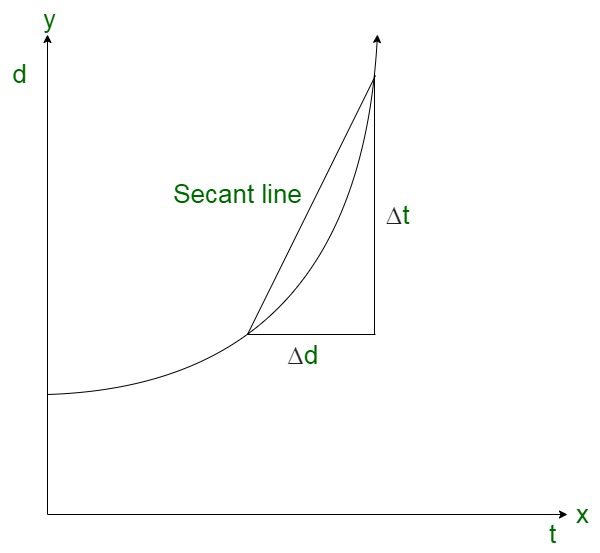Difference in Average Velocity and Average Speed

Relative Velocity

The estimation of the velocity of an object in reference to another object in the same time frame, is known as relative velocity.

Let us consider, the relative velocity of object A with respect to object B, to be

VAB = VA – VB

In case, the two objects are moving in the same direction, then

When two objects are moving in opposite direction, then

When two objects are moving at an angle, then

andExamples;

Example 1. If a car goes a distance of 900 m in the west in 90 seconds. Find the speed and velocity of car?

Solution:

Here,

Distance = 900 m

Time = 90 s

Speed =Velocity = 10 m/s to west

Example 2. If a car is moving with the uniform speed covers a distance of 240 m in 6 seconds. Find the speed of the car and the time taken to cover a distance of 480 m.

Solution:

Here,

Distance = 240 m

Time = 6 sec

Speed of train ‘v’ = ?

Time take by train to cover 480 m distance ‘t’ = ?

Speed =Time taken by the car to cover 480 m distance =Question 3. If a train travels from Delhi to Jaipur at the speed of 120 km/h and takes 3 hours to reach. Calculate the distance between the cities?

Solution:

Here

Speed of the train ‘v’ = 120 km/h

Time taken ‘t’ = 3 hours

We have to find the Distance ‘s’ = ?

Distance = Speed × Time

= 120 × 3

= 360 km

Question 4. A boy throws a ball up in the air the ball rises about 50 m vertically in 2.5 seconds, the comes back to the boy in the same position in another 2.5 seconds. Calculate

(i) Distance travelled

(ii) Displacement

(iii) Average Speed

(iv) Average Velocity

Solution:

Here,

Distance travelled upwards = 50 m

Time taken = 2.5 seconds

(i) Total distance travelled = Distance travelled upward + Distance travelled downwards

= 50 + 50

= 100 m

(ii) Displacement = As the ball reaches to its initial point hence their will be zero displacement

= 0

(iii) Average Speed =(iv) Average velocity = 0 {As the displacement is 0 thus the velocity is also 0}.

Example 5. If a car takes 4 hours to reach from Shimla to Chandigarh that has distance of 153 km from Shimla. If the car takes 5 hours for the return journey i.e. Chandigarh to Shimla. Calculate the average Speed and average Velocity of the car?

Solution:

Here,

Distance traveled in both the journeys s1, s2 = 153 km

Time taken for Shimla to Chandigarh t1 = 4 hours

Time taken for Chandigarh to Shimla t2 = 5 hours

We have to find average speed = ?

and average velocity = ?

Total Distance travelled = s1 + s2 = 153 km + 153 km = 306 km

Total Time taken = t + t = 4h + 5h = 9 h

Average Speed =Average Speed =As the car reaches back to Shimla i.e starting point thus the displacement is zero.

Thus,

Average velocity = 0

Example 6. If a train driver has the reaction time of 0.4 s between seeing the obstacle and applying the brakes. Assume the train is travelling at a speed of 72 km/h and the driver spots the obstacle calculate the distance travelled before applying the brakes?

Solution:

Here,

Reaction time ‘t’ = 0.4 s

Speed of the Train ‘v’ = 72 km/h orWe have to find the distance travelled ‘s’ = ?

Distance = speed × time

Distance = 20 × 0.4

Distance = 8 m

Example 7. Assume a ball moves with velocity v in the direction of the mirror and the mirror moves with velocity v in the direction of the ball,  So calculate the relative speed of ball’s image according to the ball?

Solution:

Velocity of the ball according to ground = v

Velocity of the mirror according to ground = v

Velocity of the ball’s image in the mirror according to = v

Image and ball both are moving towards each other so relative velocity will be = v+v = 2v.

My Personal Notes arrow_drop_up